Question

# A wire carrying a 31.0 A current bends through a right angle.

Exercise 28.13 - Enhanced - with Solution

A wire carrying a 31.0 A current bends through a right angle. Consider two 2.00 mm segments of wire, each 3.00 cm from the bend (Figure 1).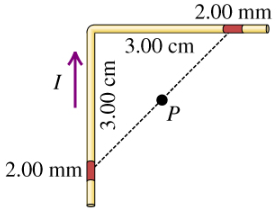Part A

Find the magnitude of the magnetic field these two segments produce at point P, which is midway between them. Express your answer with the appropriate units.

Part B

Find the direction of the magnetic field at point P.

• out of the page

• into the page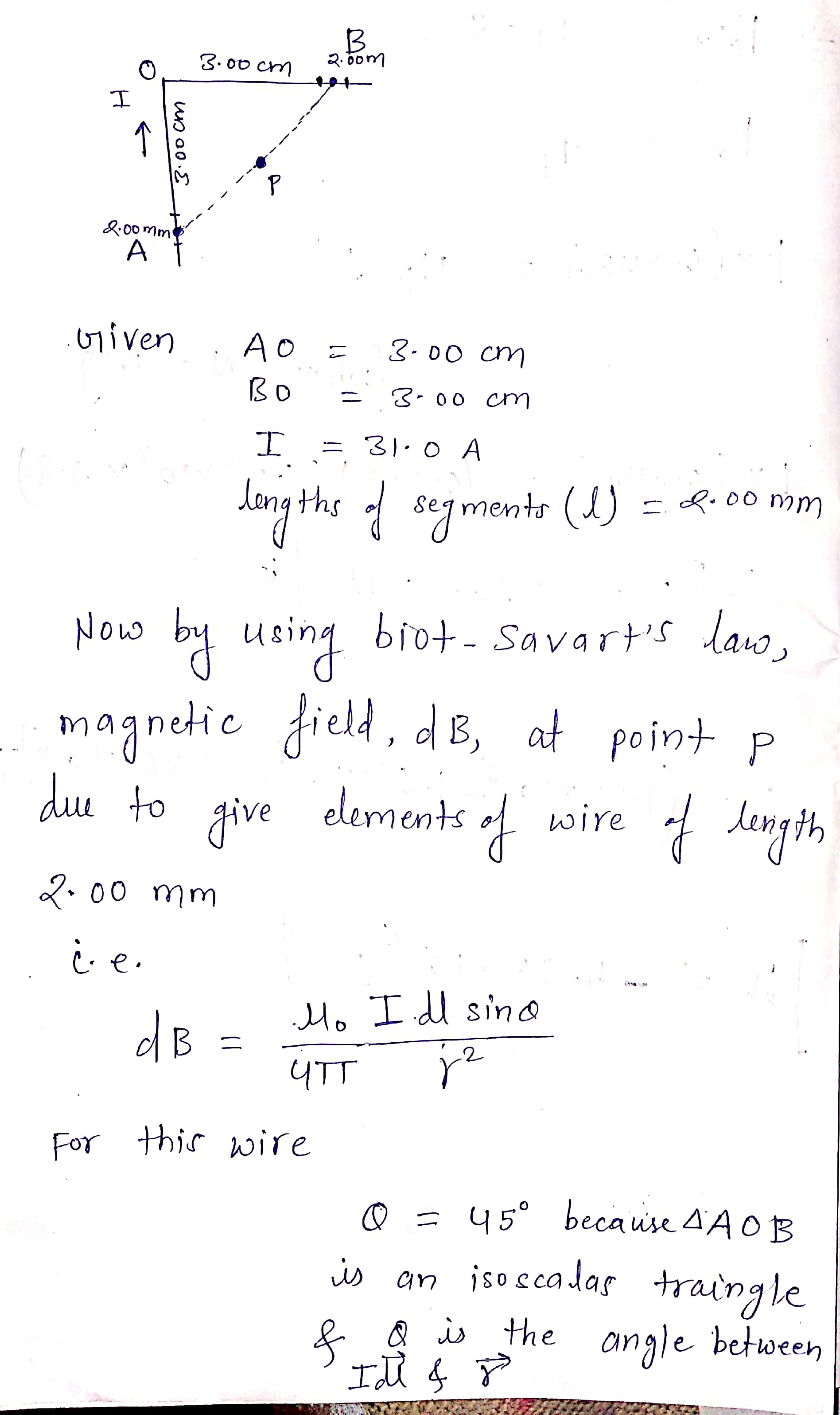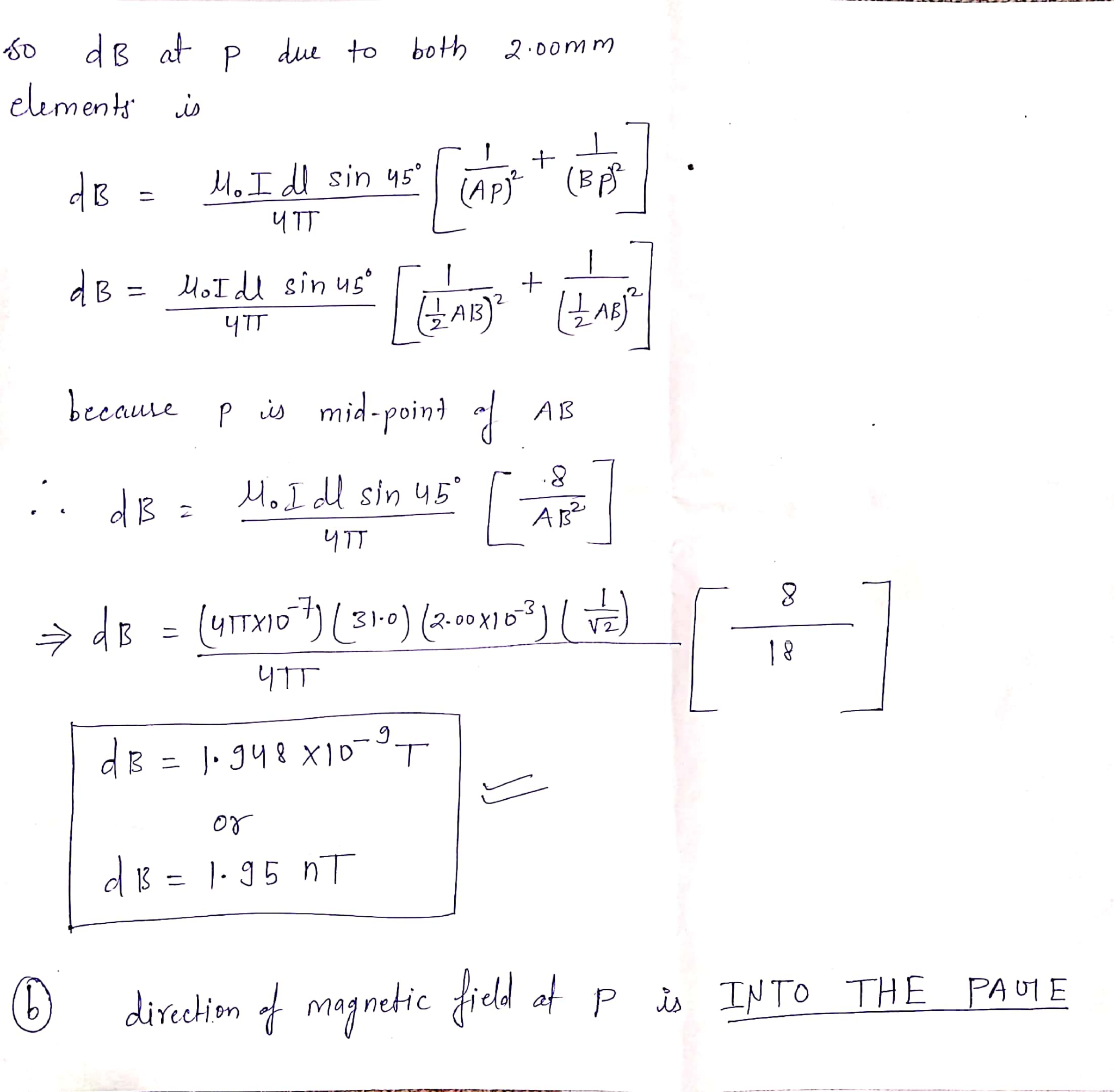#### Earn Coins

Coins can be redeemed for fabulous gifts.

Similar Homework Help Questions
• ### 4. A wire carrying a 27.0 A current bend 2.00 mm segments of wire, each 3.00...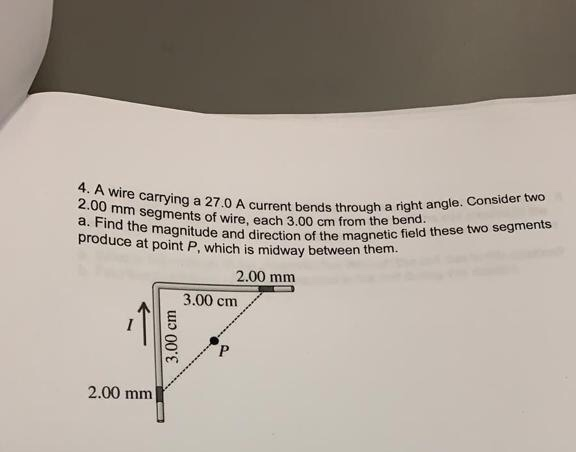4. A wire carrying a 27.0 A current bend 2.00 mm segments of wire, each 3.00 cm from a. Find the magnitude and direction produce at point P, which is midway between them rent bends through a right angle. Consider two re, each 3.00 cm from the bend. and direction of the magnetic field these two segments 2.00 mm 3.00 cm 3.00 cm 2.00 mm

• ### A long wire carrying a 6.00A current reverses direction by means of two right-angle bends, as...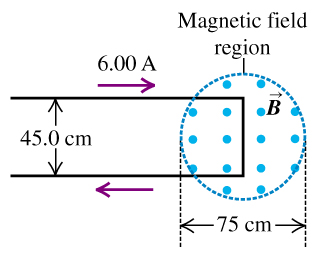A long wire carrying a 6.00A current reverses direction by means of two right-angle bends, as shown in the figure (Figure 1) . The part of the wire where the bend occurs is in a magnetic field of 0.666T confined to the circular region of diameter 75cm, as shown. A) Find the direction of the net force that the magnetic field exerts on this wire. B) Find the magnitude of the net force that the magnetic field exerts on this...

• ### A long wire carrying 4.50 A of current makes two 90 bends, as shown in the...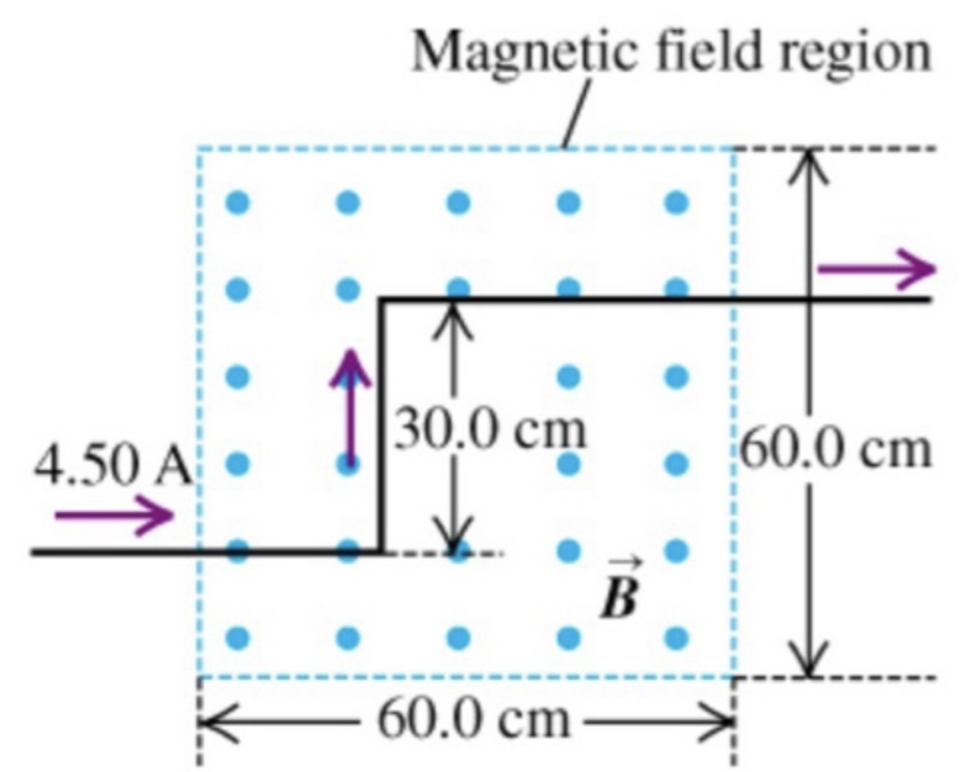A long wire carrying 4.50 A of current makes two 90° bends, as shown in the figureThe bent part of the wire passes through a uniform 0.238 T magnetic field directed as shown in the figure and confined to a limited region of space.Part AFind the magnitude of the force that the magnetic field experts on the the wire.Part BFind the direction of the force that the magnetic field experts on the the wire.

• ### A long wire carrying 6.50 A of current makes two bends, as shown in the figure.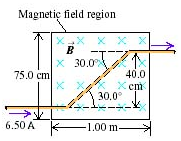A long wire carrying 6.50 A of current makes two bends, as shown in the figure. The bent part of the wire passes through a uniform 0.280 T magnetic field directed as shown in the figure and confined to a limited region of space.A) Find the magnitude of the force that the magnetic field exerts on the wire.B) Find the direction of the force that the magnetic field exerts on the wire.

• ### A long wire carrying 4.50 A of current makes two 90 degree bends, as shown in....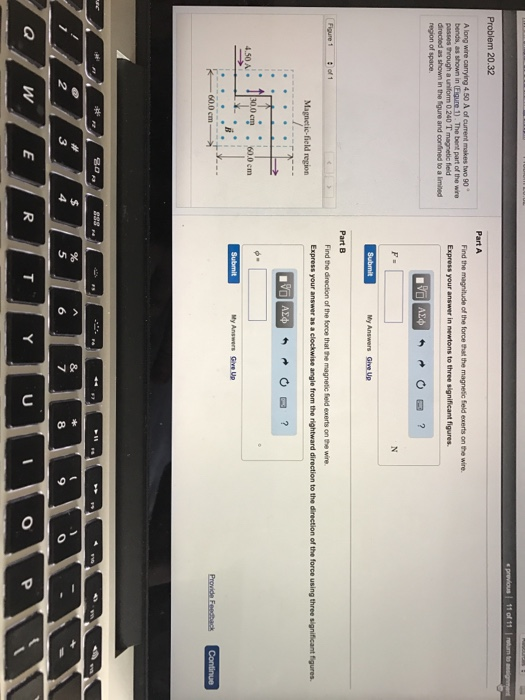A long wire carrying 4.50 A of current makes two 90 degree bends, as shown in. The best part of the wire passes through a uniform 0.240 t magnetic field directed as shown in the figure and confined to a limited of space. Part A Find the magnitude of the force that the magnetic field exerts on the wire Part B Find the direction of the force that the magnetic field exerts on the wire.

• ### ​A long wire carrying 6.50 A of currents makes two bends, as shown in the figure on the right.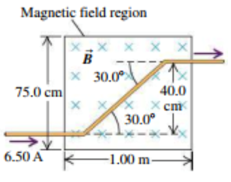A long wire carrying 6.50 A of currents makes two bends, as shown in the figure on the right. The bent part of the wire passes through a uniform 0.280-T magnetic field directed as shown and confined to a limited region of space. a. What are the magnitude and direction of the force exerted on the wire by the magnetic field?b. Now assume that the magnetic field extends 1.00 m to the left and to the right of the region shown...

• ### Consider the current-carrying wire shown in the figure. The current creates a magnetic field at the...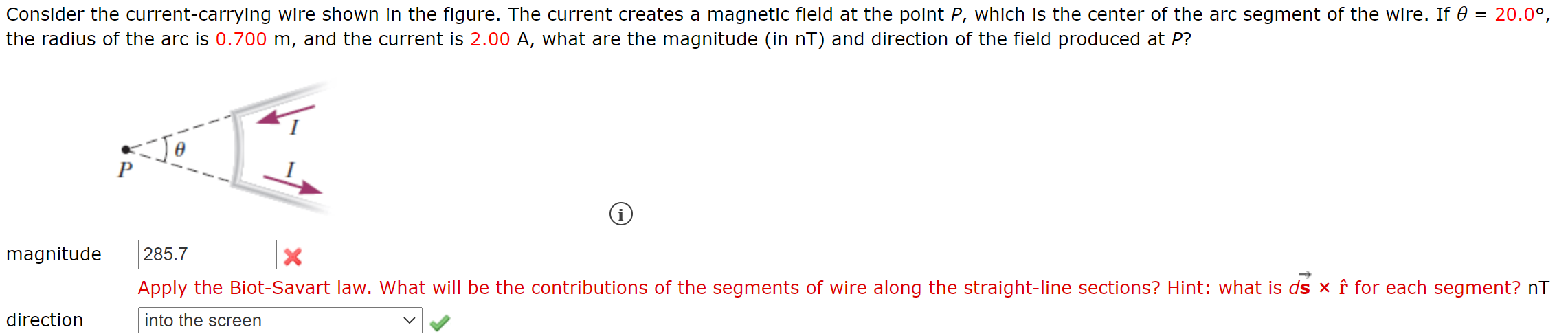Consider the current-carrying wire shown in the figure. The current creates a magnetic field at the point P, which is the center of the arc segment of the wire. If 0 = 20.0°, the radius of the arc is 0.700 m, and the current is 2.00 A, what are the magnitude (in nT) and direction of the field produced at P? P i magnitude 285.7 Apply the Biot-Savart law. What will be the contributions of the segments of wire along...

• ### l wo current-carrying wires are located 12.5 cm apart, as shown below. The wire on the...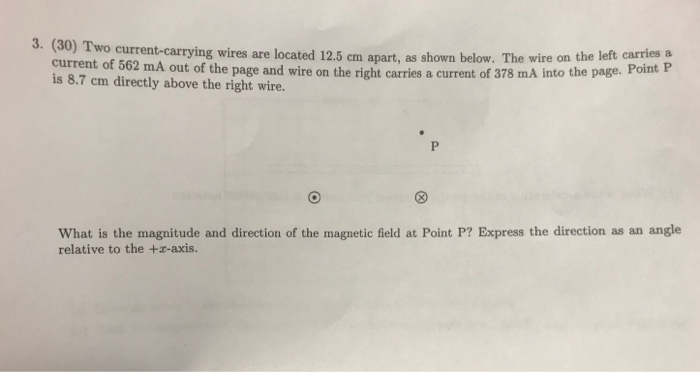l wo current-carrying wires are located 12.5 cm apart, as shown below. The wire on the left carries a current of 562 mA out of the page and wire on the right carries a current of 378 mA into the page. P is 8.7 cm directly above the right wire. What is the magnitude and direction of the magnetic field at Point P? Express the direction as an angle relative to the +z-axis.

• ### The diagram below shows a long wire with a right angle bend. A current of -3.25...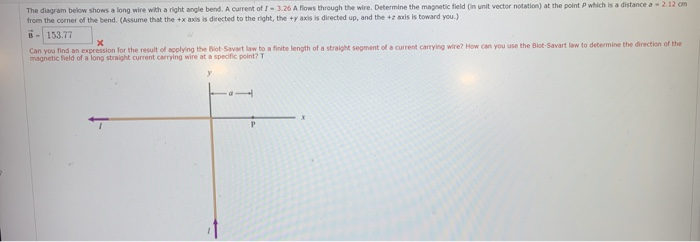The diagram below shows a long wire with a right angle bend. A current of -3.25 A flows through the wire. Determine the magnetic field in unit vector notation) at the point which is a distance - 2.12 cm from the corner of the bend. (Assume that the+xaxis is directed to the right, they axis is directed up, and the +7 xs is toward you.) B-153.77 Can you find an expression for the result of applying the Biot Savat law...